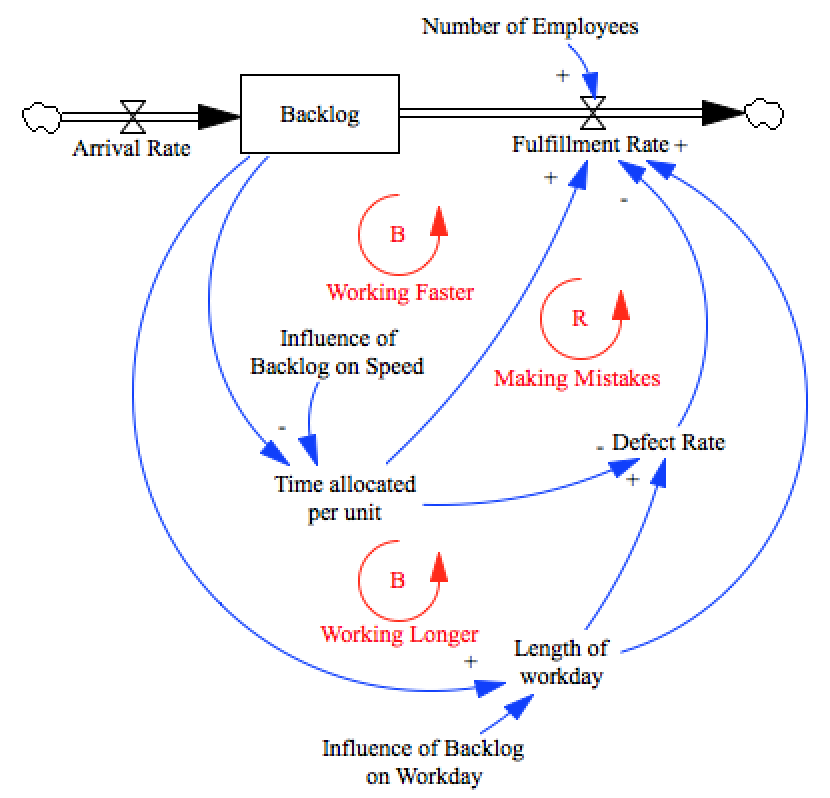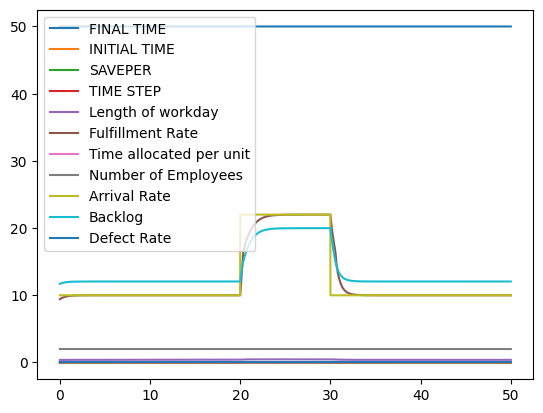# Surrogating a function with a machine learning estimator¶

System dynamics generally represents the relationships between model elements as either analytical equations, or lookup tables. However, in some situations we may be presented with relationships that are not well estimated by equations, but involve more than a single input leading to a single output. When confrontied with this situation, other paradigms%pylab inline
import pysd
import numpy as np
import pandas as pd

Populating the interactive namespace from numpy and matplotlib

/Users/houghton/anaconda/lib/python2.7/site-packages/pandas/computation/__init__.py:19: UserWarning: The installed version of numexpr 2.4.4 is not supported in pandas and will be not be used

UserWarning)

model = pysd.read_vensim('../../models/Manufacturing_Defects/Defects.mdl')

data = pd.read_csv('../../data/Defects_Synthetic/Manufacturing_Defects_Synthetic_Data.csv')

Unnamed: 0 Workday Time per Task Defect Rate
0 0 0.357563 0.036497 0.066678
1 1 0.300276 0.035329 0.063891
2 2 0.301040 0.054992 0.049828
3 3 0.290333 0.046289 0.046932
4 4 0.384306 0.050605 0.064480
plt.scatter(data['Workday'], data['Time per Task'], c=data['Defect Rate'], linewidth=0, alpha=.6)
plt.xlabel('Length of Workday')
plt.xlim(0.15, .45)
plt.ylim(.01, .09)
plt.box('off')
plt.colorbar()
plt.title('Defect Rate Measurements')
plt.figtext(.88, .5, 'Defect Rate', rotation=90, verticalalignment='center');from sklearn.svm import SVR

Outcome = data['Defect Rate'].values
regression = SVR()
regression.fit(Factors, Outcome)

SVR(C=1.0, cache_size=200, coef0=0.0, degree=3, epsilon=0.1, gamma=0.0,
kernel='rbf', max_iter=-1, shrinking=True, tol=0.001, verbose=False)

def new_defect_function():
""" Replaces the original defects equation with a regression model"""
workday = model.components.length_of_workday()

model.components.defect_rate()

0.059499757838150001

model.run().plot();